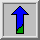# Gaussian Smoothing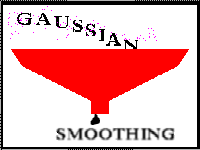Common Names: Gaussian smoothing

## Brief Description

The Gaussian smoothing operator is a 2-Dconvolution operator that is used to `blur' images andremove detail and noise. In this sense it is similar to the mean filter, but it uses a different kernel that represents the shape of a Gaussian (`bell-shaped') hump. This kernel has some special properties which are detailed below.## How It Works

The Gaussian distribution in 1-D has the form: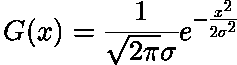where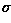is the standard deviation of the distribution. We have also assumed that the distribution has a mean of zero (i.e. it is centered on the line x=0). The distribution is illustrated in Figure 1.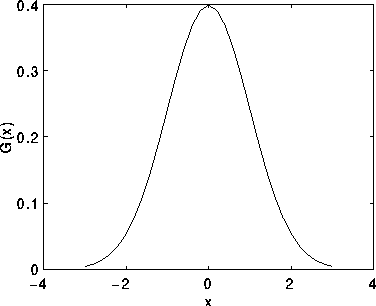Figure 1 1-D Gaussian distribution with mean 0 and=1

In 2-D, an isotropic (i.e. circularly symmetric) Gaussian has the form: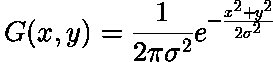This distribution is shown in Figure 2.Figure 2 2-D Gaussian distribution with mean (0,0) and=1

The idea of Gaussian smoothing is to use this 2-D distribution as a `point-spread' function, and this is achieved by convolution. Since the image is stored as a collection of discrete pixels we need to produce a discrete approximation to the Gaussian function before we can perform the convolution. In theory, the Gaussian distribution is non-zero everywhere, which would require an infinitely large convolution kernel, but in practice it is effectively zero more than about three standard deviations from the mean, and so we can truncate the kernel at this point. Figure 3 shows a suitable integer-valued convolution kernel that approximates a Gaussian with aof 1.0. It is not obvious how to pick the values of the mask to approximate a Gaussian. One could use the value of the Gaussian at the centre of a pixel in the mask, but this is not accurate because the value of the Gaussian varies non-linearly across the pixel. We integrated the value of the Gaussian over the whole pixel (by summing the Gaussian at 0.001 increments). The integrals are not integers: we rescaled the array so that the corners had the value 1. Finally, the 273 is the sum of all the values in the mask.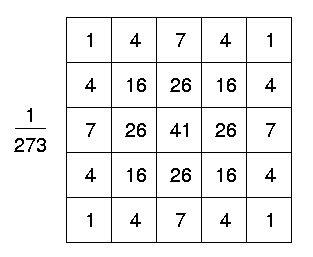Figure 3 Discrete approximation to Gaussian function with=1.0

Once a suitable kernel has been calculated, then the Gaussian smoothing can be performed using standard convolution methods. The convolution can in fact be performed fairly quickly since the equation for the 2-D isotropic Gaussian shown above is separable into x and y components. Thus the 2-D convolution can be performed by first convolving with a 1-D Gaussian in the x direction, and then convolving with another 1-D Gaussian in the y direction. (The Gaussian is in fact the only completely circularly symmetric operator which can be decomposed in such a way.) Figure 4 shows the 1-D x component kernel that would be used to produce the full kernel shown in Figure 3 (after scaling by 273, rounding and truncating one row of pixels around the boundary because they mostly have the value 0. This reduces the 7x7 matrix to the 5x5 shown above.). The y component is exactly the same but is oriented vertically.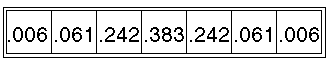Figure 4 One of the pair of 1-D convolution kernels used to calculate the full kernel shown in Figure 3 more quickly.

A further way to compute a Gaussian smoothing with a large standard deviation is to convolve an image several times with a smaller Gaussian. While this is computationally complex, it can have applicability if the processing is carried out using a hardware pipeline.

The Gaussian filter not only has utility in engineering applications. It is also attracting attention from computational biologists because it has been attributed with some amount of biological plausibility, e.g. some cells in the visual pathways of the brain often have an approximately Gaussian response.## Guidelines for Use

The effect of Gaussian smoothing is to blur an image, in a similar fashion to the mean filter. The degree of smoothing is determined by the standard deviation of the Gaussian. (Larger standard deviation Gaussians, of course, require larger convolution kernels in order to be accurately represented.)

The Gaussian outputs a `weighted average' of each pixel's neighborhood, with the average weighted more towards the value of the central pixels. This is in contrast to the mean filter's uniformly weighted average. Because of this, a Gaussian provides gentler smoothing and preserves edges better than a similarly sized mean filter.

One of the principle justifications for using the Gaussian as a smoothing filter is due to itsfrequency response. Most convolution-based smoothing filters act aslowpass frequency filters. This means that their effect is to remove high spatial frequency components from an image. The frequency response of a convolution filter, i.e. its effect on different spatial frequencies, can be seen by taking theFourier transform of the filter. Figure 5 shows the frequency responses of a 1-D mean filter with width 5 and also of a Gaussian filter with= 3.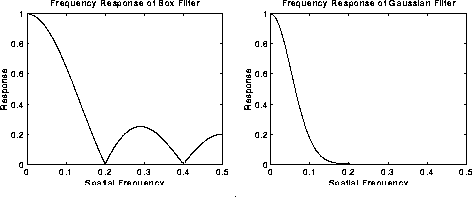Figure 5 Frequency responses of Box (i.e. mean) filter (width 5 pixels) and Gaussian filter (= 3 pixels). The spatial frequency axis is marked in cycles per pixel, and hence no value above 0.5 has a real meaning.

Both filters attenuate high frequencies more than low frequencies, but the mean filter exhibits oscillations in its frequency response. The Gaussian on the other hand shows no oscillations. In fact, the shape of the frequency response curve is itself (half a) Gaussian. So by choosing an appropriately sized Gaussian filter we can be fairly confident about what range of spatial frequencies are still present in the image after filtering, which is not the case of the mean filter. This has consequences for some edge detection techniques, as mentioned in the section onzero crossings. (The Gaussian filter also turns out to be very similar to the optimal smoothing filter for edge detection under the criteria used to derive the Canny edge detector.)

We use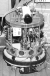to illustrate the effect of smoothing with successively larger and larger Gaussian filters.

The image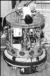shows the effect of filtering with a Gaussian of= 1.0 (and kernel size 5×5).

The image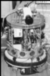shows the effect of filtering with a Gaussian of= 2.0 (and kernel size 9×9).

The image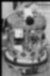shows the effect of filtering with a Gaussian of= 4.0 (and kernel size 15×15).

We now consider using the Gaussian filter for noise reduction. For example, consider the imagewhich has been corrupted by Gaussian noise with a mean of zero and= 8. Smoothing this with a 5×5 Gaussian yields(Compare this result with that achieved by the mean and median filters.)

Salt and pepper noise is more challenging for a Gaussian filter. Here we will smooth the imagewhich has been corrupted by 1% salt and pepper noise (i.e. individual bits have been flipped with probability 1%). The imageshows the result of Gaussian smoothing (using the same convolution as above). Compare this with the originalNotice that much of the noise still exists and that, although it has decreased in magnitude somewhat, it has been smeared out over a larger spatial region. Increasing the standard deviation continues to reduce/blur the intensity of the noise, but also attenuates high frequency detail (e.g. edges) significantly, as shown inThis type of noise is better reduced using median filtering, conservative smoothing or Crimmins Speckle Removal.

## Interactive Experimentation

You can interactively experiment with this operator by clicking here.

## Exercises

1. Starting from the Gaussian noise (mean 0,= 13) corrupted imagecompute both mean filter and Gaussian filter smoothing at various scales, and compare each in terms of noise removal vs loss of detail.

2. At how many standard deviations from the mean does a Gaussian fall to 5% of its peak value? On the basis of this suggest a suitable square kernel size for a Gaussian filter with= s.

3. Estimate the frequency response for a Gaussian filter by Gaussian smoothing an image, and taking its Fourier transform both before and afterwards. Compare this with the frequency response of a mean filter.

4. How does the time taken to smooth with a Gaussian filter compare with the time taken to smooth with a mean filter for a kernel of the same size? Notice that in both cases the convolution can be speeded up considerably by exploiting certain features of the kernel.

## References

E. Davies Machine Vision: Theory, Algorithms and Practicalities, Academic Press, 1990, pp 42 - 44.

R. Gonzalez and R. Woods Digital Image Processing, Addison-Wesley Publishing Company, 1992, p 191.

R. Haralick and L. Shapiro Computer and Robot Vision, Addison-Wesley Publishing Company, 1992, Vol. 1, Chap. 7.

B. Horn Robot Vision, MIT Press, 1986, Chap. 8.

D. Vernon Machine Vision, Prentice-Hall, 1991, pp 59 - 61, 214.

## Local Information